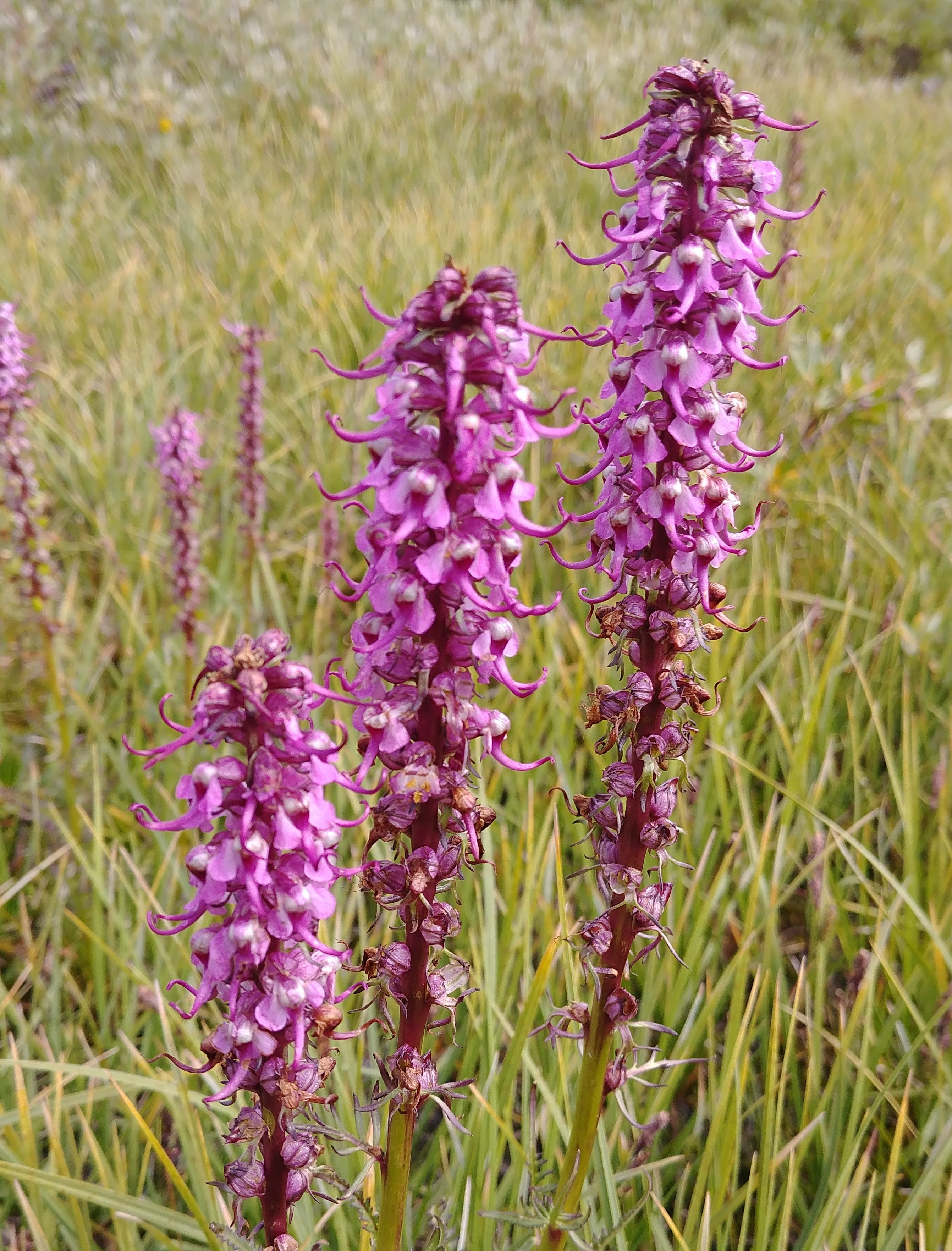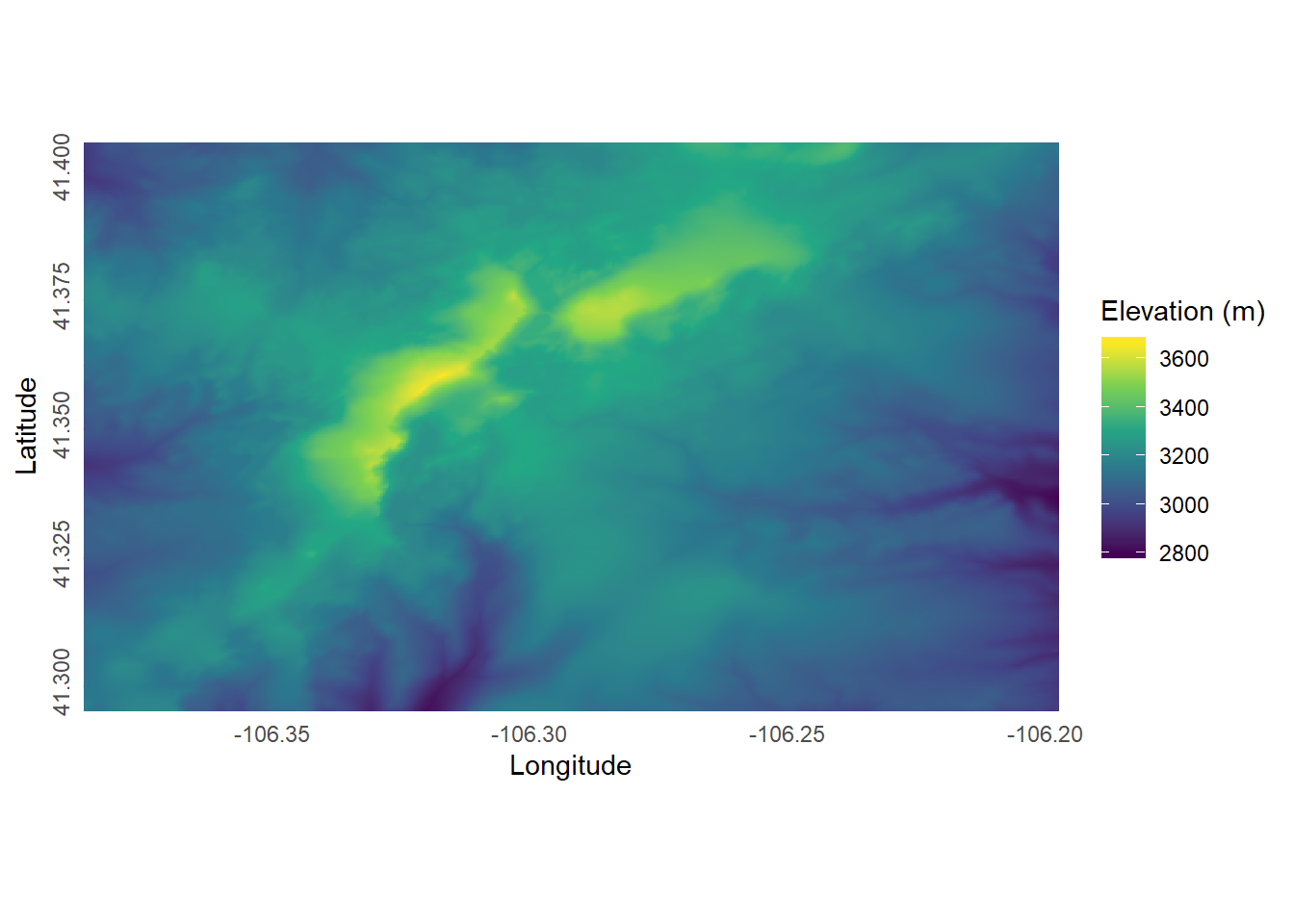# Wetlands and landscapes

Data science, water, isotopes, and ecosystems

## Using rayshader on a DEM of the Snowy Range

Exploring html widget functionalities with rayshader.In this post I’m hoping to test out some of the html widget capabilities of using blogdown with Hugo in the form of a dynamic graph made with rayshader and rgl R packages. The end result should be a DEM of the Snowy Range in Wyoming, US that you can zoom in on, rotate, and otherwise manipulate.

### Libraries

First, let’s load some of the libraries that we’ll need.

``````library(rgl)
``## Warning: package 'rayshader' was built under R version 3.6.2``
``library(raster)``
``## Warning: package 'raster' was built under R version 3.6.2``
``````library(sp)
library(tidyverse)``````
``## Warning: package 'tidyverse' was built under R version 3.6.2``
``## Warning: package 'tidyr' was built under R version 3.6.2``
``## Warning: package 'dplyr' was built under R version 3.6.2``

Now let’s load a USGS DEM that covers an area of the Snowy Range that is elevationally interesting (covers Medicine Bow Peak, Brown’s Peak, and Sugarloaf).

``````#Load in the USGS raster that I'm going to use.
"/floatn42w107.tif")
snowy_raw <- raster(tif_loc)
common_crs <- crs(snowy_raw)
#Clip the raster, because we don't need all of it.
# These are just eyeball estimates.
clip_box_df <- tibble(place = c("upper-left", "upper-right", "lower-left",
"lower-right"),
x = c(-106.386531, -106.197495, -106.386531, -106.197495),
y = c(41.405525, 41.405525, 41.295257, 41.295257))
clip_box <- clip_box_df
coordinates(clip_box) <- ~ x + y
proj4string(clip_box) <- as.character(common_crs)
snowy <- crop(snowy_raw, clip_box)
#Convery to dataframe for nicer plotting with ggplot.
snowy_df <- snowy %>%
as.data.frame(xy = TRUE) %>%
rename(z = floatn42w107)``````

### Static visualization

Alright, now let’s see what this clipped DEM looks like.

``````ggplot(snowy_df) +
geom_raster(aes(x, y, fill = z)) +
scale_fill_viridis_c() +
labs(x = "Longitude",
y = "Latitude",
fill = "Elevation (m)") +
coord_equal() +
theme_minimal() +
theme(axis.text.y = element_text(angle = 90, hjust = 0.43)) +
scale_x_continuous(expand = c(0, 0)) +
scale_y_continuous(expand = c(0, 0))``````Nice. Now let’s see what the rayshader graphic looks like.

### Dynamic visualization

This visual output from this render process can be pretty large (> 60 MB), so it may take a hot second to load.

``````#Convert the Snowy Range raster to a matrix.
snowy_mat = matrix(
data = raster::extract(snowy, raster::extent(snowy),
buffer = 1500),
nrow = ncol(snowy),
ncol = nrow(snowy)
)

#Initialize some of the rayshader inputs.
z_scale <- 8 #Stretching out the elevations for a bit of visual appeal.
snowy_norm <- calculate_normal(snowy_mat, zscale = z_scale)
snowy_amb <- ambient_shade(snowy_mat, zscale = z_scale)

#Visualize!
snowy_mat %>%